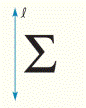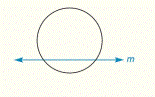Chapter 2.CR, Problem 46CRElementary Geometry For College St...

7th Edition
Alexander + 2 others
ISBN: 9781337614085

Solutions

Chapter
SectionElementary Geometry For College St...

7th Edition
Alexander + 2 others
ISBN: 9781337614085
Textbook Problem

Complete the drawing so that the figure is reflected across a) line l b) line mTo determine

a)

To draw:

The line of reflection across the line 𝓁.

Explanation

Given:

The given figure is,

Figure (1)

Property used:

With the reflection, every point of the original figure is reflected across a line in such a way as to make the given line a line of symmetry. Each pair of corresponding points will lie on opposite sides of the line of reflection and at equal distances.

Approach:

The drawing so that the Figure (1) is reflected across the line

To determine

(b)

To draw:

The line of reflection across the line m.

Still sussing out bartleby?

Check out a sample textbook solution.

See a sample solution

The Solution to Your Study Problems

Bartleby provides explanations to thousands of textbook problems written by our experts, many with advanced degrees!

Get Started

In Exercises 49-62, find the indicated limit, if it exists. 50. limx2x24x+2

Applied Calculus for the Managerial, Life, and Social Sciences: A Brief Approach

Find the distance between the points. 1. (1, 1), (4, 5)

Single Variable Calculus: Early Transcendentals, Volume I

Show that if an 0 and limn nan 0, then an is divergent.

Single Variable Calculus: Early Transcendentals

True or False: dmdv stands for the derivative of the function m with respect to the variable v.

Study Guide for Stewart's Single Variable Calculus: Early Transcendentals, 8th